## 7. The Poincaré disk model for the hyperbolic plane

The second model that we use to represent the hyperbolic plane is called the Poincaré disk model, named after the great French mathematician, Henri Poincaré (1854 - 1912).

This model is constructed starting from the previous one. You may ask yourself why is it necessary to do that? The reason is that this model is more useful, as it gives more insight and we can make a better use of the apparatus from the complex analysis. As you will see at the end of this page, it is this model that appears in Escher's work.

The underlying space of this model is the open unit disk. Note that we are still in the complex plane.

Just like in the half-plane model, we will look first at lines in this model.

### Hyperbolic Lines

Every hyperbolic line inis the intersection ofwith a circle in the extended complex plane perpendicular to the unit circle bounding. Moreover, every such intersection is a hyperbolic line.

Remember that in the half-plane case, the lines were either Euclidean lines, perpendicular onto the real line, or half-circles, also perpendicular onto the real line. In the Poincaré case, lines are given by diameters of the circle or arcs. Here is a figure to give you an idea: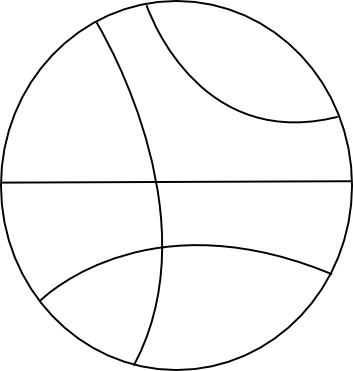### Distances in hyperbolic plane

One quantity that we can calculate in Euclidean geometry is the distance between two points, which is given by the absolute value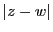, ifandare the two points, considered in the complex plane. In the upper half-plane model it is very difficult to express this Euclidean distanceto the hyperbolic distance between a given pair of points. One useful feature of the Poincaré disk model is that there does exist such an easily expressed relationship between the Euclidean and hyperbolic distance.

Property 1   For each pairandof points inwe have that: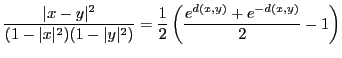where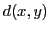is the hyperbolic distance between the two points.

This tells us that it's pretty complicated to calculate the hyperbolic distance in, but nevertheless it is much easier than in the half-space case.

Since we can calculate distances, we can now define circles and polygons, and we can calculate areas.

### Hyperbolic circles

Definition 1   A hyperbolic circle inis a set of the form: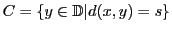whereis the hyperbolic center of the circle, andis the hyperbolic radius (so they are fixed).

We can completely characterise hyperbolic circles in. Here are some properties:

Property 2   A hyperbolic circle inis a Euclidean circle inand vice-versa, although the hyperbolic and Euclidean centers, and the hyperbolic and Euclidean radii, generally don't coincide.

Property 3   Given, let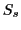be the hyperbolic circle inwith hyperbolic center 0 and hyperbolic radius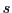The hyperbolic length ofis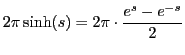(Remember, in the Euclidean the circumference is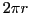, where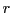is the radius).

Note that any hyperbolic circle is inside the Poincaré disk, so from the Euclidean point of view any circle here has radius less than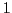!

Now that you have these formulas, you can try to calculate some hyperbolic radii. Here are some exercises:

Exercise 1   Let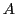be the Euclidean circle in the Poincaré disk with Euclidean center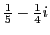and Euclidean radius. Determine the hyperbolic center and the hyperbolic radius.

Exercise 2   Letbe a circle with Euclidean centerand radius. Show that the hyperbolic center isand the hyperbolic radius satisfies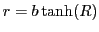, where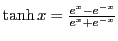.

### Hyperbolic polygons

In Euclidean geometry, the polygon is one of the basic geometric objects. It is a closed convex set, bounded by Euclidean lines. We can view it as an intersection of a collection of half-planes. This is the basis for our definition.

Definition 2   A hyperbolic polygon is a closed convex set in the hyperbolic plane, that can be expressed as the intersection of a (locally finite) collection of closed half-planes.

(What locally finite means is a bit complicated to explain, it requires some deep analysis. But in principle it means that if we would enlarge (with a good enough microscope) the intersections between neighbouring half-planes, there will always be finitely many planes which intersect).

Here is an example. It is the intersection of four closed half-planes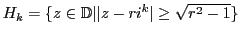, for.### Hyperbolic fish and other beings...

Like we said before, the Poincaré disk model is very good to visualise objects. Thanks to Escher we can see how "beings" (which are in fact more complicated 2-D objects) look in the hyperbolic plane. Here is again "Circle Limit III" and this time with its original sketch.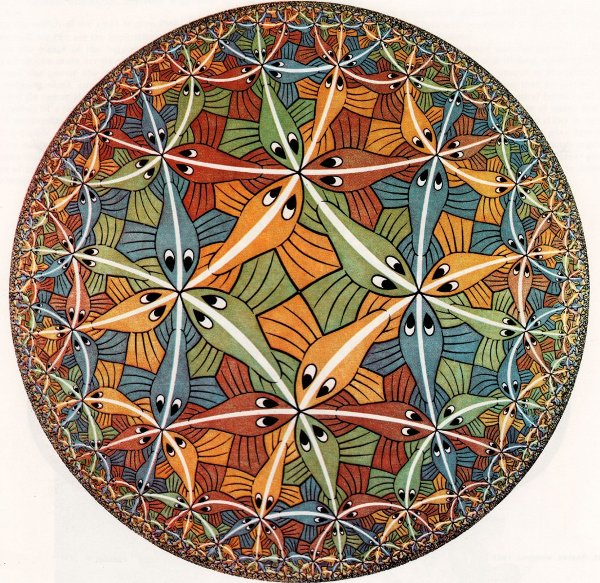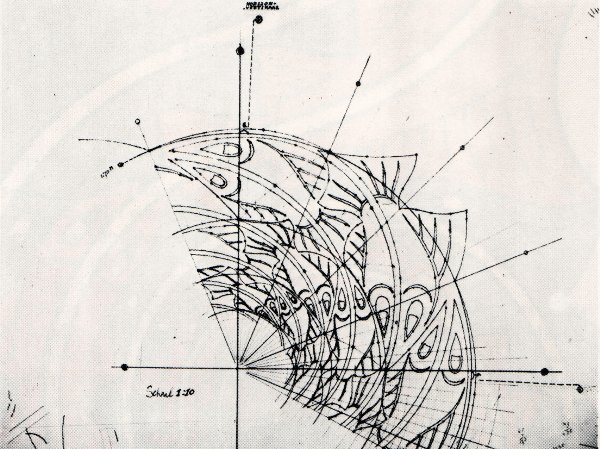"Circle Limit III" "Circle Limit III - original sketch"

Although the fish appear smaller the closer you get to the boundary of the disk, they are the same size in the geometry of the hyperbolic plane. Similarly, all the white segments going from the fishes' tails to their noses are the same length (and they are, in fact, straight lines). This tells us that, in a way, we can imagine this plane as a kind of sphere, that has a point at infinity.

"Circle Limit III" is not just a representation of the Poncaré disk in a more artistic way, it is also a tessellation of the hyperbolic. Tessellations were Escher's favourite game! Let's see what they are!

 INDEX NEXT